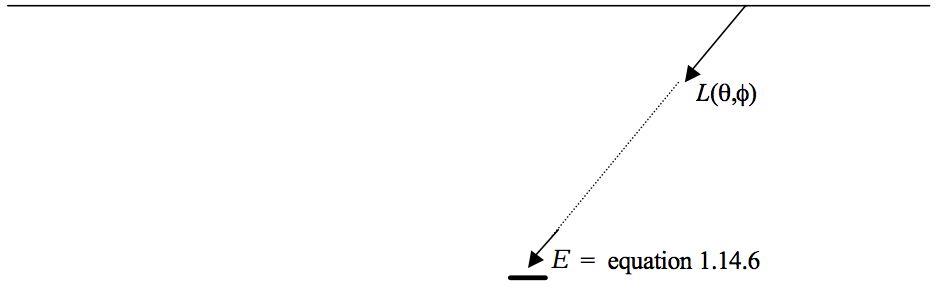$$\require{cancel}$$

# 1.14: Relations between Flux, Intensity, Exitance, Irradiance

•• Contributed by Jeremy Tatum
• Emeritus Professor (Physics & Astronomy) at University of Victoria

In this section I am going to ask, and answer, three questions.

i. (See figure I.3 )$$\text{FIGURE I.3}$$

A point source of light has an intensity that varies with direction as $$I(\theta , \phi$$). What is the radiant flux radiated into the hemisphere $$\theta < \pi /2$$? This is easy; we already answered it for a complete sphere in equation 1.6.3. For a hemisphere, the answer is

$\phi = \int_0^{2\pi} \int_0^{\pi/2} I(\theta, \phi) \sin \theta d \theta d \phi. \tag{1.14.1} \label{1.14.1}$

ii. At a certain point on an extended plane radiating surface, the radiance is $$L(\theta ,\phi$$). What is the emergent exitance $$M$$ at that point?$$\text{FIGURE I.4}$$

Consider an elemental area $$\delta A$$ (see figure I.4). The intensity $$I( \theta , \phi$$) radiated in the direction $$(\theta ,\phi )$$ is the radiance times the projected area $$\cos \theta \ \delta A$$. Therefore the radiant power or flux radiated by the element into the hemisphere is

$\delta \phi = \int_0^{2\pi} \int_0^{\pi/2} L(\theta, \phi) \cos \theta \sin \theta d\theta d \phi \delta A, \tag{1.14.2} \label{1.14.2}$

and therefore the exitance is

$M=\int_0^{2\pi} \int_0^{\pi/2} L(\theta, \phi) \cos \theta \sin \theta d\theta d\phi \tag{1.14.3} \label{1.14.3}$

iii. A point $$O$$ is at the centre of the base of a hollow radiating hemisphere whose radiance in the direction $$(\theta , \phi )$$ is $$L(\theta , \phi)$$. What is the irradiance at that point $$O$$ ? (See figure I.5.)$$\text{FIGURE I.5}$$

Consider an elemental area $$a^2 \ \sin \theta \ \delta \theta \ \delta\phi$$ on the inside of the hemisphere at a point where the radiance is $$L(\theta ,\phi )$$ (figure I.5). The intensity radiated towards $$O$$ is the radiance times the area:

$\delta I (\theta, \phi) = L(\theta, \phi) a^2 \sin \theta \delta \theta \delta \phi \tag{1.14.4} \label{1.14.4}$

The irradiance at $$O$$ from this elemental area is (see equation (1.10.1)

$\delta E = \frac{\delta I (\theta,\phi) \cos \theta}{a^2} = L (\theta, \phi) \cos \theta \sin \theta \delta \theta \delta \phi, \tag{1.14.5} \label{1.14.5}$

and so the irradiance at O from the entire hemisphere is

$E = \int_0^{2\pi} \int_0^{\pi/2} L(\theta, \phi) \cos \theta \sin \theta \delta \theta \delta \phi. \tag{1.14.6} \label{1.14.6}$

The same would apply for any shape of inverted bowl - or even an infinite plane radiating surface (see figure I.6.)$$\text{FIGURE I.6}$$